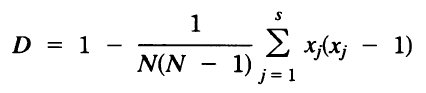# Discriminatory Power Calculator

 The Discriminatory Power (D) is the average probability that the typing system will assign a different type to two unrelated strains randomly sampled in the microbial population of a given taxon. This tool has been created to help calculate this parameter

 The Discriminatory Power (D), as shown by Hunter can be expressed by the formula of Simpson´s index of diversity, which reads:Where D is the index of discriminatory power, N the number of unrelated strains tested, S the number of different types, and xj the number of strains belonging to the jth type, assuming that strains will be classified into mutually exclusive categories. Thus, a D value of 1.0 would indicate that a typing method was able to distinguish each member of a strain population from all other members of that population. Conversely, an index of 0.0 would indicate that all members of a strain population were of an identical type. An index of 0.50 would mean that if one strain was chosen at random from a strain population, then there would be a 50% probability that the next strain chosen at random would be indistinguishable from the first. References: Hunter P. Reproducibility and indices of discriminatory power of microbial typing methods. J Clin Microbiol 1990; 28: 1903-5. PubMed Hunter PR and Gaston MA. Numerical index of the discriminatory ability of typing systems: an application of Simpson´s index of diversity. J Clin Microbiol. 1988 November; 26(11): 2465–2466. PubMed Start using this tool